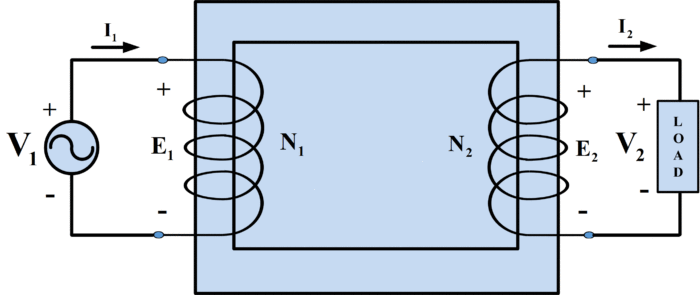Home / Transformer / Transformer Working Principle | How Transformer Works

# Transformer Working Principle | How Transformer Works

Want create site? Find Free WordPress Themes and plugins.

## Transformer Definition

A device consisting of two or more windings coupled by a magnetic core that is used to transform a balanced set of three-phase voltages from one voltage level to another without changing the frequency.

The transformer is an essential element of an electrical power system. It is among the primary reasons for the far-flung utilization of AC power systems. It makes power generation possible at the most efficient voltage, transmission system and distribution at the most economic voltage levels, and electric power usage at the most appropriate voltage. The electric transformer is also extensively applied to measure very high voltages using voltage or potential transformers and very large currents using a current transformer). Additional salient uses of transformers include impedance matching, Insulating one electric circuit from another.

## Transformer Working Principle

A single-phase transformer fundamentally comprises two main windings coupled up by a magnetic core. When one of the windings (common refer as primary) is linked to an AC power source, a time-varying flux is generated in the core which links the second winding (commonly referred as secondary winding). Consequently, a voltage is induced in the secondary winding. When an electrical load is connected to the secondary winding, a secondary current starts flowing.

A single-phase transformer unit is exemplified in Fig 1. The primary and secondary windings have N1 and N2 turns respectively. The voltages and currents associated with each winding are stated in the pharos form.Fig.1: A transformer circuit

Ideal transformer Characteristics

An ideal transformer is characterized by the following:

1.     There is NO flux leakage which means that the fluxes associated with the primary and secondary currents are restricted within the core.

2.     Primary and Secondary windings have no resistances which mean that the applied voltage (source voltage) v1 is same as the induced primary voltage e1; that is, v1 = e1. Similarly, v2 = e2

3.     The magnetic core has an infinite permeability which means that the reluctance of the core is zero. Hence, a very small amount of current is needed to set up the magnetic flux.

4.     The magnetic core is lossless which means that hysteresis, as well as eddy current losses, are insignificant.

## How Transformer Works

Let the mutual flux, which is linking both windings, be sinusoidal, that is,

$\begin{matrix} {{\phi }_{m}}={{\Phi }_{m}}\sin \omega t & {} & (1) \\\end{matrix}$

Then, according to Faraday’s law of electromagnetic induction. The induced emf’s may be expressed as

$\begin{matrix} {{e}_{1}}=\frac{d{{\lambda }_{1}}}{dt}={{N}_{1}}\frac{d{{f}_{m}}}{dt}=\omega {{\Phi }_{p}}{{N}_{1}}\cos \omega t & {} & (2) \\\end{matrix}$

$\begin{matrix} {{e}_{2}}=\frac{d{{\lambda }_{2}}}{dt}={{N}_{2}}\frac{d{{f}_{m}}}{dt}=\omega {{\Phi }_{p}}{{N}_{2}}\cos \omega t~ & {} & (3) \\\end{matrix}$

The rms values of the induced voltages are

$\begin{matrix} {{E}_{1}}=\frac{1}{\sqrt{2}}\omega {{\Phi }_{p}}{{N}_{1}}=4.44f{{\Phi }_{p}}{{N}_{1}} & {} & (4) \\\end{matrix}$

$\begin{matrix} {{E}_{2}}=\frac{1}{\sqrt{2}}\omega {{\Phi }_{p}}{{N}_{2}}=4.44f{{\Phi }_{p}}{{N}_{2}} & {} & (5) \\\end{matrix}$

Where $f={\omega }/{2\pi }\;$  cycles per second or hertz.

The induced voltages polarities are specified by Lenz’s law; that is, the emf’s produce currents that tend to oppose the change in flux. The ratio of the induced voltages can be written as:

$\begin{matrix} \frac{{{E}_{1}}}{{{E}_{2}}}=\frac{{{N}_{1}}}{{{N}_{2}}}=a & {} & (6) \\\end{matrix}$

Where a is called the turns ratio, since the transformer is ideal, the induced voltages are equal to their corresponding terminal voltages; that is, E1 = V1 and E2 = V2. Therefore,

$\begin{matrix} \frac{{{E}_{1}}}{{{E}_{2}}}=\frac{{{V}_{1}}}{{{V}_{2}}}=a & {} & (7) \\\end{matrix}$

The postulation that the magnetic circuit of an ideal transformer is lossless indicates that the mmfs produced by the windings balance or cancel each other; that is, primary mmf equals secondary mmf. In terms of the winding currents, this may be stated as

$\begin{matrix} {{N}_{1}}{{I}_{1}}={{N}_{2}}{{I}_{2}} & {} & (8) \\\end{matrix}$

Equation (8) demonstrates that the winding currents are in phase with each other and that their magnitudes are related by

$\begin{matrix} \frac{{{I}_{1}}}{{{I}_{2}}}=\frac{{{N}_{2}}}{{{N}_{1}}}=\frac{1}{a} & {} & (9) \\\end{matrix}$

The primary voltage and current may be expressed in terms of their secondary counterparts as follows:

$\begin{matrix} {{V}_{1}}=a{{V}_{2}} & {} & (10) \\\end{matrix}$

$\begin{matrix} {{I}_{1}}=\left( \frac{1}{a} \right){{I}_{2}} & {} & (11) \\\end{matrix}$

Multiplying equations (10) and (11) yields

$\begin{matrix} {{V}_{1}}{{I}_{1}}={{V}_{2}}{{I}_{2}} & {} & (12) \\\end{matrix}$

Equation (12) states the power invariance law across the ideal transformer. In other words, the input power to the transformer is equal to its output power.

Dividing equation (10) by (11) provides the relation for the secondary impedance referred to the primary side:

$\begin{matrix} \frac{{{V}_{1}}}{{{I}_{1}}}=\frac{{{a}^{2}}{{V}_{2}}}{{{I}_{2}}} & {} & (13) \\\end{matrix}$

$\begin{matrix} {{Z}_{1}}={{a}^{2}}{{Z}_{2}} & {} & (14) \\\end{matrix}$

The equivalent circuit of an ideal transformer is illustrated in Fig.2, with all quantities referred to the same side.Fig.2 (a):   Equivalent circuit of an ideal transformer (a) all quantities referred to primary sideFig.2 (b):   Equivalent circuit of an ideal transformer (a) all quantities referred to secondary side

## Transformer Calculations Example

A 60-Hz ideal transformer is rated 220/110 V. an inductive load Z2 = 10 + j10 Ω is connected across the low-voltage side at rated secondary voltage. Calculate the following:

1. Primary and secondary currents
2. Load impedance referred to the primary
3. Power supplied by the source

Solution

1. The turns ratio is

$a=\frac{{{V}_{1}}}{{{V}_{2}}}=\frac{220}{110}=2$

The primary and secondary currents are found as follows:

${{\text{I}}_{\text{2}}}=\frac{{{V}_{2}}}{{{Z}_{2}}}=\frac{110\angle {{0}^{o}}}{10+j10}=7.78\angle -{{45}^{o}}A$

${{\text{I}}_{1}}=\left( \frac{1}{a} \right){{\text{I}}_{2}}=\left( \frac{1}{2} \right)7.78\angle -{{45}^{o}}=3.89\angle -{{45}^{o}}A$

2.The load impedance referred to the primary side is

${{Z}_{1}}={{a}^{2}}{{Z}_{2}}={{\left( 2 \right)}^{2}}(10+j10)=40+j40\Omega$

3. The power supplied by the source is computed as follows:

${{P}_{1}}={{P}_{2}}={{V}_{2}}{{I}_{2}}=(110)(7.78)cos{{45}^{o}}=605W$

${{P}_{1}}={{P}_{2}}={{I}^{2}}{{R}_{2}}={{(7.78)}^{2}}(10)=605W$

The performance of a transformer is described in terms of its voltage regulation and its efficiency. The transformer performance can be predicted from the results of two tests known as the open-circuit test and short-circuit test.

Did you find apk for android? You can find new Free Android Games and apps.